Pulkit Sharma — Updated On June 20th, 2022

## Introduction

When I started my deep learning journey, one of the first things I learned was image classification. It’s such a fascinating part of the computer vision fraternity and I was completely immersed in it! But I have a curious mind and once I had a handle on image classification, I wondered if I could transfer that learning to videos.

Was there a way to build a model that automatically identified specific people in a given video at a particular time interval? Turns out, there was and I’m excited to share my approach with you!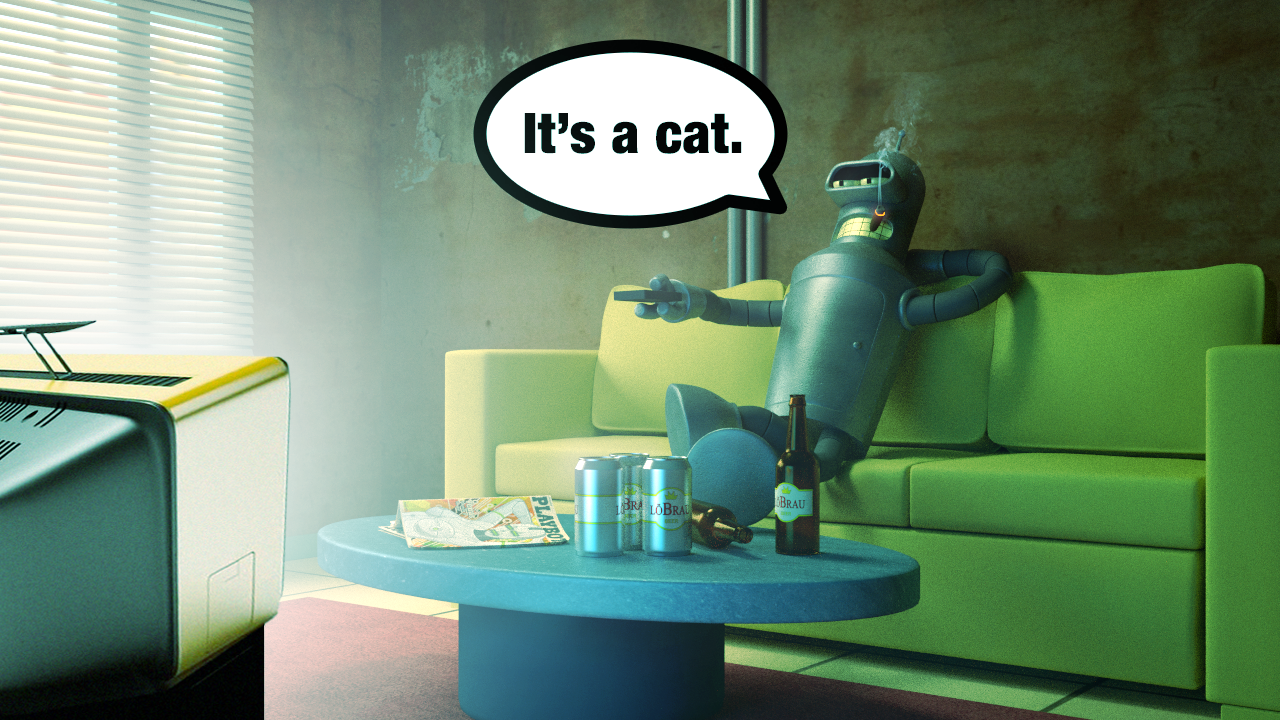Source: Coastline Automation

Now to give you some context on the problem we’ll be solving, keep in mind that screen time is extremely important for an actor. It is directly related to the money he/she gets. Just to give you a sense of this commission, did you know that Robert Downey Jr. Downey picked up \$10 million for just 15 minutes of screen time in “Spider-Man Homecoming”? Incredible.

How cool would it be if we could take any video and calculate the screen time of any actor present in it?

In this article, I will help you understand how to use deep learning on video data. To do this, we will be working with videos from the popular TOM and JERRY cartoon series. The aim is to calculate the screen time of both TOM and JERRY in any given video.

Note: This article assumes you have a prior knowledge of image classification using deep learning. If not, I recommend going through this article which will help you get a grasp of the basics of deep learning and image classification.

1. Reading a video and extracting frames
2. How to handle video files in Python
3. Calculating the screen time – A simple Solution
4. My learnings – what worked and what did not

## Reading a video and extracting frames

Ever heard of a flip book? If you haven’t, you’re missing out! Check out the one below: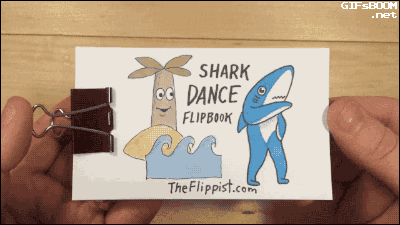(Source: giphy.com)

We have a different image on each page of the book, and as we flip these pages, we get an animation of a shark dancing. You could even call it a kind of video. The visualization gets better the  faster we flip the pages. In other words, this visual is a collection of different images arranged in a particular order.

Similarly, videos are nothing but a collection of a set of images. These images are called frames and can be combined to get the original video. So, a problem related to video data is not that different from an image classification or an object detection problem. There is just one extra step of extracting frames from the video.

Remember, our challenge here is to calculate the screen time of both Tom and Jerry from a given video. Let me first summarize the steps we will follow in this article to crack this problem:

1. Import and read the video, extract frames from it, and save them as images
2. Label a few images for training the model (Don’t worry, I have done it for you)
3. Build our model on training data
4. Make predictions for the remaining images
5. Calculate the screen time of both TOM and JERRY

Believe me, just following these steps will help you in solving many such video related problems in deep learning. Time to get our Python hats on now, and dig into this challenge.

## How to handle video files in Python

```import cv2     # for capturing videos
import math   # for mathematical operations
import matplotlib.pyplot as plt    # for plotting the images
%matplotlib inline
import pandas as pd
from keras.preprocessing import image   # for preprocessing the images
import numpy as np    # for mathematical operations
from keras.utils import np_utils
from skimage.transform import resize   # for resizing images
```

### Step – 1: Read the video, extract frames from it and save them as images

Now we will load the video and convert it into frames. You can download the video used for this example from this link. We will first capture the video from the given directory using the VideoCapture() function, and then we’ll extract frames from the video and save them as an image using the imwrite() function. Let’s code it:

Python Code:

Once this process is complete, ‘Done!’ will be printed on the screen as confirmation that the frames have been created.

Let us try to visualize an image (frame). We will first read the image using the imread() function of matplotlib, and then plot it using the imshow() function.

```img = plt.imread('frame0.jpg')   # reading image using its name
plt.imshow(img)```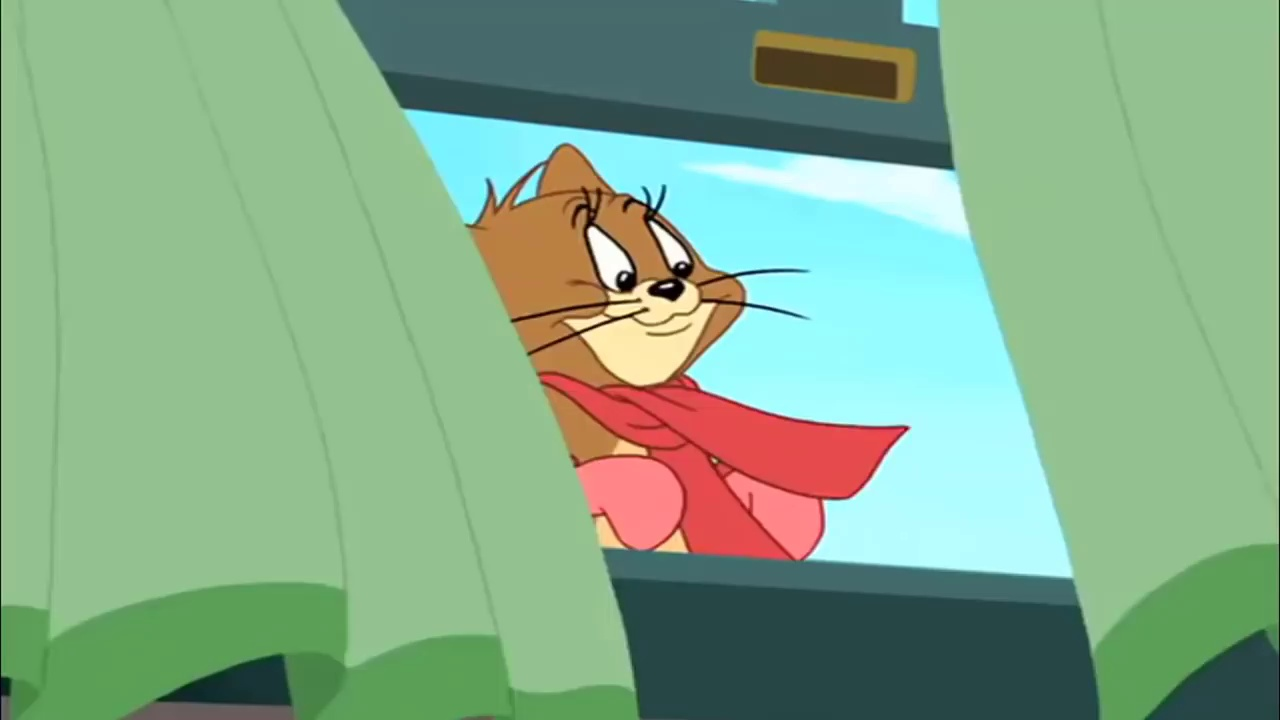Getting excited, yet?

This is the first frame from the video. We have extracted one frame for each second, from the entire duration of the video. Since the duration of the video is 4:58 minutes (298 seconds), we now have 298 images in total.

Our task is to identify which image has TOM, and which image has JERRY. If our extracted images would have been similar to the ones present in the popular Imagenet dataset, this challenge could have been a breeze. How? We could simply have used models pre-trained on that Imagenet data and achieved a high accuracy score! But then where’s the fun in that?

We have cartoon images so it’ll be very difficult (if not impossible) for any pre-trained model to identify TOM and JERRY in a given video.

### Step – 2: Label a few images for training the model

So how do we go about handling this? A possible solution is to manually give labels to a few of the images and train the model on them. Once the model has learned the patterns, we can use it to make predictions on a previously unseen set of images.

Keep in mind that there could be frames when neither TOM nor JERRY are present. So, we will treat it as a multi-class classification problem. The classes which I have defined are:

• 0 – neither JERRY nor TOM
• 1 – for JERRY
• 2 – for TOM

Don’t worry, I have labelled all the images so you don’t have to! Go ahead and download the mapping.csv file which contains each image name and their corresponding class (0 or 1 or 2).

```data = pd.read_csv('mapping.csv')     # reading the csv file
data.head()      # printing first five rows of the file```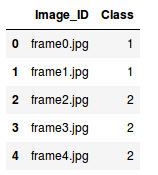The mapping file contains two columns:

• Image_ID: Contains the name of each image
• Class: Contains corresponding class for each image

Our next step is to read the images which we will do based on their names, aka, the Image_ID column.

```X = [ ]     # creating an empty array
for img_name in data.Image_ID:
X.append(img)  # storing each image in array X
X = np.array(X)    # converting list to array```

Tada! We now have the images with us. Remember, we need two things to train our model:

• Training images, and
• Their corresponding class

Since there are three classes, we will one hot encode them using the to_categorical() function of keras.utils.

```y = data.Class
dummy_y = np_utils.to_categorical(y)    # one hot encoding Classes```

We will be using a VGG16 pretrained model which takes an input image of shape (224 X 224 X 3). Since our images are in a different size, we need to reshape all of them. We will use the resize() function of skimage.transform to do this.

```image = []
for i in range(0,X.shape):
a = resize(X[i], preserve_range=True, output_shape=(224,224)).astype(int)      # reshaping to 224*224*3
image.append(a)
X = np.array(image)```

All the images have been reshaped to 224 X 224 X 3. But before passing any input to the model, we must preprocess it as per the model’s requirement. Otherwise, the model will not perform well enough. Use the preprocess_input() function of keras.applications.vgg16 to perform this step.

```from keras.applications.vgg16 import preprocess_input
X = preprocess_input(X, mode='tf')      # preprocessing the input data```

We also need a validation set to check the performance of the model on unseen images. We will make use of the train_test_split() function of the sklearn.model_selection module to randomly divide images into training and validation set.

```from sklearn.model_selection import train_test_split
X_train, X_valid, y_train, y_valid = train_test_split(X, dummy_y, test_size=0.3, random_state=42)    # preparing the validation set```

### Step 3: Building the model

The next step is to build our model. As mentioned, we shall be using the VGG16 pretrained model for this task. Let us first import the required libraries to build the model:

```from keras.models import Sequential
from keras.applications.vgg16 import VGG16
from keras.layers import Dense, InputLayer, Dropout```

We will now load the VGG16 pretrained model and store it as base_model:

`base_model = VGG16(weights='imagenet', include_top=False, input_shape=(224, 224, 3))    # include_top=False to remove the top layer`

We will make predictions using this model for X_train and X_valid, get the features, and then use those features to retrain the model.

```X_train = base_model.predict(X_train)
X_valid = base_model.predict(X_valid)
X_train.shape, X_valid.shape```

The shape of X_train and X_valid is (208, 7, 7, 512), (90, 7, 7, 512) respectively. In order to pass it to our neural network, we have to reshape it to 1-D.

```X_train = X_train.reshape(208, 7*7*512)      # converting to 1-D
X_valid = X_valid.reshape(90, 7*7*512)```

We will now preprocess the images and make them zero-centered which helps the model to converge faster.

```train = X_train/X_train.max()      # centering the data
X_valid = X_valid/X_train.max()```

Finally, we will build our model. This step can be divided into 3 sub-steps:

1. Building the model
2. Compiling the model
3. Training the model
```# i. Building the model
model = Sequential()

Let’s check the summary of the model using the summary() function:

`model.summary()`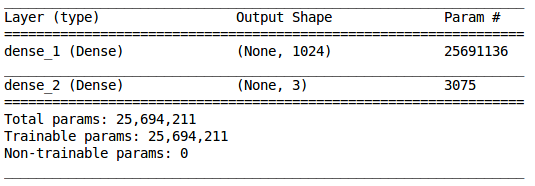We have a hidden layer with 1,024 neurons and an output layer with 3 neurons (since we have 3 classes to predict). Now we will compile our model:

```# ii. Compiling the model

In the final step, we will fit the model and simultaneously also check its performance on the unseen images, i.e., validation images:

```# iii. Training the model
model.fit(train, y_train, epochs=100, validation_data=(X_valid, y_valid))```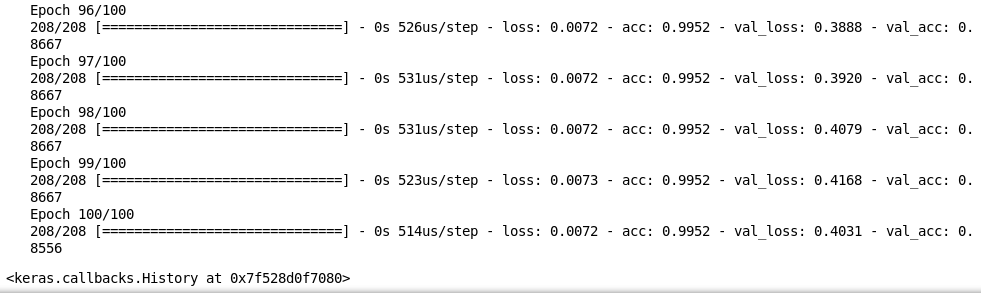We can see it is performing really well on the training as well as the validation images. We got an accuracy of around 85% on unseen images. And this is how we train a model on video data to get predictions for each frame.

In the next section, we will try to calculate the screen time of TOM and JERRY in a new video.

## Calculating the screen time – A simple solution

First, download the video we’ll be using in this section from here. Once done, go ahead and load the video and extract frames from it. We will follow the same steps as we did above:

```count = 0
videoFile = "Tom and Jerry 3.mp4"
cap = cv2.VideoCapture(videoFile)
frameRate = cap.get(5) #frame rate
x=1
while(cap.isOpened()):
frameId = cap.get(1) #current frame number
if (ret != True):
break
if (frameId % math.floor(frameRate) == 0):
filename ="test%d.jpg" % count;count+=1
cv2.imwrite(filename, frame)
cap.release()
print ("Done!")```

Done!

After extracting the frames from the new video, we will now load the test.csv file which contains the names of each extracted frame. Download the test.csv file and load it:

`test = pd.read_csv('test.csv')`

Next, we will import the images for testing and then reshape them as per the requirements of the aforementioned pretrained model:

```test_image = []
for img_name in test.Image_ID:
test_image.append(img)
test_img = np.array(test_image)```
```test_image = []
for i in range(0,test_img.shape):
a = resize(test_img[i], preserve_range=True, output_shape=(224,224)).astype(int)
test_image.append(a)
test_image = np.array(test_image)

```

We need to make changes to these images similar to the ones we did for the training images. We will preprocess the images, use the base_model.predict() function to extract features from these images using the VGG16 pretrained model, reshape these images to 1-D form, and make them zero-centered:

```# preprocessing the images
test_image = preprocess_input(test_image, mode='tf')

# extracting features from the images using pretrained model
test_image = base_model.predict(test_image)

# converting the images to 1-D form
test_image = test_image.reshape(186, 7*7*512)

# zero centered images
test_image = test_image/test_image.max()```

Since we have trained the model previously, we will make use of that model to make prediction for these images.

### Step – 4: Make predictions for the remaining images

`predictions = model.predict_classes(test_image)`

### Step – 5 Calculate the screen time of both TOM and JERRY

Recall that Class ‘1’ represents the presence of JERRY, while Class ‘2’ represents the presence of TOM. We shall make use of the above predictions to calculate the screen time of both these legendary characters:

```print("The screen time of JERRY is", predictions[predictions==1].shape, "seconds")
print("The screen time of TOM is", predictions[predictions==2].shape, "seconds")```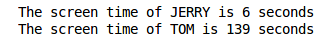And there you go! We have the total screen time of both TOM and JERRY in the given video.

## My learnings – what worked and what did not

I tried and tested many things for this challenge – some worked exceedingly well, while some ended up flat. In this section, I will elaborate a bit on some of the difficulties I faced, and then how I tackled them. After that, I have provided the entire code for the final model which gave me the best accuracy.

First, I tried using the pretrained model without removing the top layer. The results were not satisfactory. The possible reason could be that these are the cartoon images and our pretrained model was trained on actual images and hence it was not able to classify these cartoon images. To tackle this problem, i retrained the pretrain model using few labelled images and the results were better from the previous results.

Even after training on the labelled images, the accuracy was not satisfactory. The model was not able to perform well on the training images itself. So, i tried to increase the number of layers. Increasing the number of layers proved to be a good solution to increase the training accuracy but there was no sync between training and validation accuracy. The model was overfitting and its performance on the unseen data was not satisfactory. So I added a Dropout layer after every Dense layer and then there was good sync between training and validation accuracy.

I noticed that the classes are imbalanced. TOM had more screen time so the predictions were dominated by it and most of the frames were predicted as TOM. To overcome this and make the classes balanced, i used compute_class_weight() function of sklearn.utils.class_weight module. It assigned higher weights to the classes with lower value counts as compared to the classes with higher value counts.

I also used Model Checkpointing to save the best model, i.e. the model which produced lowest validation loss and then used that model to make the final predictions. I will summarize all the above mentioned steps and will give the final code now. The actual classes for the testing images can be found in testing.csv file.

```import cv2
import math
import matplotlib.pyplot as plt
import pandas as pd
%matplotlib inline
from keras.preprocessing import image
import numpy as np
from skimage.transform import resize```
```count = 0
videoFile = "Tom and jerry.mp4"
cap = cv2.VideoCapture(videoFile)
frameRate = cap.get(5) #frame rate
x=1
while(cap.isOpened()):
frameId = cap.get(1) #current frame number
if (ret != True):
break
if (frameId % math.floor(frameRate) == 0):
filename ="frame%d.jpg" % count;count+=1
cv2.imwrite(filename, frame)
cap.release()
print ("Done!")```

Done!

```count = 0
videoFile = "Tom and Jerry 3.mp4"
cap = cv2.VideoCapture(videoFile)
frameRate = cap.get(5) #frame rate
x=1
while(cap.isOpened()):
frameId = cap.get(1) #current frame number
if (ret != True):
break
if (frameId % math.floor(frameRate) == 0):
filename ="test%d.jpg" % count;count+=1
cv2.imwrite(filename, frame)
cap.release()
print ("Done!")```

Done!

```data = pd.read_csv('mapping.csv')
```X = []
for img_name in data.Image_ID:
X.append(img)
X = np.array(X)```
```test_image = []
for img_name in test.Image_ID:
test_image.append(img)
test_img = np.array(test_image)```
```from keras.utils import np_utils
train_y = np_utils.to_categorical(data.Class)
test_y = np_utils.to_categorical(test.Class)```
```image = []
for i in range(0,X.shape):
a = resize(X[i], preserve_range=True, output_shape=(224,224,3)).astype(int)
image.append(a)
X = np.array(image)```
```test_image = []
for i in range(0,test_img.shape):
a = resize(test_img[i], preserve_range=True, output_shape=(224,224)).astype(int)
test_image.append(a)
test_image = np.array(test_image)```
```from keras.applications.vgg16 import preprocess_input
X = preprocess_input(X, mode='tf')
test_image = preprocess_input(test_image, mode='tf')```
```from sklearn.model_selection import train_test_split
X_train, X_valid, y_train, y_valid = train_test_split(X, train_y, test_size=0.3, random_state=42)```
```from keras.models import Sequential
from keras.applications.vgg16 import VGG16
from keras.layers import Dense, InputLayer, Dropout```
`base_model = VGG16(weights='imagenet', include_top=False, input_shape=(224, 224, 3))`
```X_train = base_model.predict(X_train)
X_valid = base_model.predict(X_valid)
test_image = base_model.predict(test_image)```
```X_train = X_train.reshape(208, 7*7*512)
X_valid = X_valid.reshape(90, 7*7*512)
test_image = test_image.reshape(186, 7*7*512)```
```train = X_train/X_train.max()
X_valid = X_valid/X_train.max()
test_image = test_image/test_image.max()```
```model = Sequential()
`model.compile(loss='categorical_crossentropy', optimizer='adam', metrics=['accuracy'])`
```from sklearn.utils.class_weight import compute_class_weight, compute_sample_weight
class_weights = compute_class_weight('balanced',np.unique(data.Class), data.Class)  # computing weights of different classes```
```from keras.callbacks import ModelCheckpoint
filepath="weights.best.hdf5"
checkpoint = ModelCheckpoint(filepath, monitor='val_loss', verbose=1, save_best_only=True, mode='min')
callbacks_list = [checkpoint]      # model check pointing based on validation loss```
`model.fit(train, y_train, epochs=100, validation_data=(X_valid, y_valid), class_weight=class_weights, callbacks=callbacks_list)`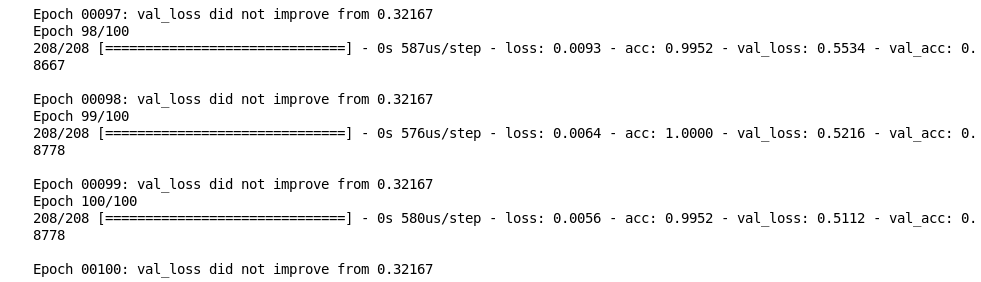`model.load_weights("weights.best.hdf5")`
`model.compile(loss='categorical_crossentropy', optimizer='adam', metrics=['accuracy'])`
```scores = model.evaluate(test_image, test_y)
print("%s: %.2f%%" % (model.metrics_names, scores*100))```

## Conclusion

We got an accuracy of around 88% on the validation data and 64% on the test data using this model.

One possible reason for getting a low accuracy on test data could be a lack of training data. As the model does not have much knowledge of cartoon images like TOM and JERRY, we must feed it more images during the training process. My advice would be to extract more frames from different TOM and JERRY videos, label them accordingly, and use them for training the model. Once the model has seen a plethora of images of these two characters, there’s a good chance it will lead to a better classification result.

Such models can help us in various fields:

• We can calculate the screen time of a particular actor in a movie
• Calculate the screen time of your favorite superhero, etc.

These are just a few examples where this technique can be used. You can come up with many more such applications on your own! Feel free to share your thoughts and feedback in the comments section below.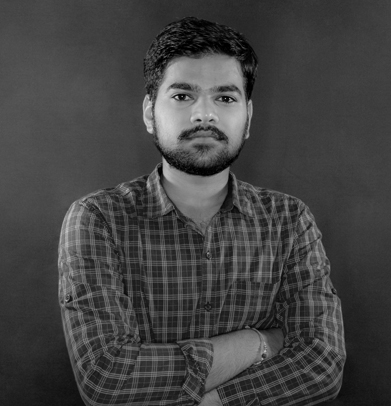###### Pulkit Sharma

My research interests lies in the field of Machine Learning and Deep Learning. Possess an enthusiasm for learning new skills and technologies.

## 45 thoughts on "Deep Learning Tutorial to Calculate the Screen Time of Actors in any Video (with Python codes)"###### Abdul Haq Syed says:September 11, 2018 at 1:02 pm###### Pulkit Sharma says:September 11, 2018 at 2:21 pm###### Konan Jean-Claude Kouassi says:September 11, 2018 at 8:19 pm
Good job Pulkit! I think it is a useful project too. But the limit is the fact that we have generate each time images from a movie and label them. We have so to build a new model for each actor. Perhaps it is good to think now on automatic models, which are generalizable on any movie (autolabelled). Reply###### Pulkit Sharma says:September 12, 2018 at 11:33 am
Hi, As per my knowledge, I don't think there are pretrained models trained on the faces of actors (correct me if I am wrong). So, we need to give labels for training the model. As seen in this project, labeling only few images can produce good results. Will look forward and try to automate these labeling part. If you find some insights related to this, please share it here. It would be helpful to take this forward. Reply###### Steve Voorhees says:September 12, 2018 at 10:45 pm
I really enjoyed this project. Thanks for your work and sharing it! I am not sure, but it looks as if a fourth category of both Tom and Jerry being in a frame is overlooked? Reply###### Pulkit Sharma says:September 13, 2018 at 11:15 am
Hi, Just for simplicity I have ignored it for now. We can treat it as multi class multi label problem to solve this fourth category. Reply###### Pabitra says:September 15, 2018 at 10:05 pm###### Pulkit Sharma says:September 17, 2018 at 12:10 pm###### tarun says:September 28, 2018 at 6:34 pm###### Pulkit Sharma says:October 03, 2018 at 11:46 am
Hi tarun, If you followed the same code given in the article, then the frames will be saved in the same directory as that of your notebook. Reply###### AKMAL ALAMGIR says:October 03, 2018 at 7:59 pm
Hi Pulkit, Thanks for the amazing stuff, while trying to resize the image in 224*224*3, i am getting index error : index 298 is out of bounds for axis 0 with size 298. What could be the possible cause and solution for the same. Thanks Reply###### Pulkit Sharma says:October 03, 2018 at 8:03 pm
Hi Akmal, What is the range that you have given in the for loop while resizing the frames? Reply###### Shrikant Pandey says:October 04, 2018 at 12:54 am
Hello @PULKIT SHARMA. I am doing this case study but i got an error. At this line of code i get error from keras.applications.vgg16 import preprocess_input X = preprocess_input(X, mode='tf') # preprocessing the input data The error comes : --------------------------------------------------------------------------- TypeError Traceback (most recent call last) in () 1 from keras.applications.vgg16 import preprocess_input ----> 2 X = preprocess_input(X, mode='tf') # preprocessing the input data ~Anaconda3libsite-packageskerasapplicationsimagenet_utils.py in preprocess_input(x, data_format, mode) 173 174 if isinstance(x, np.ndarray): --> 175 return _preprocess_numpy_input(x, data_format=data_format, mode=mode) 176 else: 177 return _preprocess_symbolic_input(x, data_format=data_format, ~Anaconda3libsite-packageskerasapplicationsimagenet_utils.py in _preprocess_numpy_input(x, data_format, mode) 40 """ 41 if mode == 'tf': ---> 42 x /= 127.5 43 x -= 1. 44 return x TypeError: ufunc 'true_divide' output (typecode 'd') could not be coerced to provided output parameter (typecode 'l') according to the casting rule ''same_kind'' Please tell me where to make change Reply###### Pulkit Sharma says:October 04, 2018 at 10:43 am
Hi Shrikant, If you want to cast the float result to an integer, you can call true_divide with out=x and casting='unsafe' arguments. Reply###### Shrikant Pandey says:October 06, 2018 at 12:37 am
Thanks for reply. Suppose i want do this case study that a videos has duration of 1 hour then how to label the frame data.Any automatic system can help or manual operation for label the frame data. Reply###### Pulkit Sharma says:October 08, 2018 at 11:16 am
Hi Shrikant, You have to label a few frames manually and then you can build your model to predict the classes for remaining frames. In the meantime, you can also look for some automated labeling platforms and share with us if you find something. Reply###### Marc Cohen says:October 12, 2018 at 2:22 pm
Hello Pulkit, Thank you for this very usefull tutorial. I have been doing the case and I am trying few things to have a better understanding of how it works. I used the "predict" function on the test images to get the probabilites of each images to be in the classes instead of just have the predicted class. It returns an array with 3 probabilites for each images but I don't understand because for all those images the sum of the probabilities is far from 1 : [2.0654954e-05 7.3857354e-03 1.3992348e-01] 0.14732987 [1.7211305e-05 3.4042433e-02 3.3835460e-02] 0.0678951 [0.002029 0.03743717 0.00029298] 0.039759144 Here is the 3 first images, with the probabilities associated to each class and the sum of those ones beside. Is it normal ? Regards, Marc Reply###### Pulkit Sharma says:October 12, 2018 at 5:08 pm
Hi Marc, Yes, it is normal. Further, in the output layer, you can use softmax activation function instead of sigmoid activation function to get probabilities for each of the 3 classes. We use softmax activation function when we have more than 2 classes. Reply###### Nikhil Konijeti says:October 15, 2018 at 6:42 pm
Hey Pulkit, This article was really helpful for me to learn a lot. I am getting an error running the below statements test_image = base_model.predict(test_image) test_image = test_image.reshape(186, 7*7*512) saying this, Error: test_image = base_model.predict(test_image) Traceback (most recent call last): File "", line 1, in test_image = base_model.predict(test_image) File "/home/nikhilkonijeti/anaconda3/envs/py35/lib/python2.7/site-packages/keras/engine/training.py", line 1147, in predict x, _, _ = self._standardize_user_data(x) File "/home/nikhilkonijeti/anaconda3/envs/py35/lib/python2.7/site-packages/keras/engine/training.py", line 749, in _standardize_user_data exception_prefix='input') File "/home/nikhilkonijeti/anaconda3/envs/py35/lib/python2.7/site-packages/keras/engine/training_utils.py", line 127, in standardize_input_data 'with shape ' + str(data_shape)) ValueError: Error when checking input: expected input_1 to have 4 dimensions, but got array with shape (0, 1) test_image = test_image.reshape(186, 7*7*512) Traceback (most recent call last): File "", line 1, in test_image = test_image.reshape(186, 7*7*512) ValueError: cannot reshape array of size 0 into shape (186,25088) Thank you in advance. Reply###### Pulkit Sharma says:October 15, 2018 at 8:00 pm
Hi Nikhil, Seems like you have not loaded the pre-trained model properly. I would suggest to rerun the entire code, run this line "test_image = base_model.predict(test_image)" and then print the shape of test_image. It should be (7,7,512). Reply###### Nikhil Konijeti says:October 16, 2018 at 9:15 pm
Thanks for your reply, Even after trying to rerun the entire code and then executing the command hasn't got to any improvement Reply###### Nikhil Konijeti says:October 16, 2018 at 10:58 pm
Thank you, got the output Reply###### ibrahim says:November 05, 2018 at 8:30 pm
Hello thanks for sharing your work, it is really insightful. why do you use the table with defined classes for the testing data set in the final code (testing.csv) , where it makes sense for me that this table is the output of the predictions. correct me if i am wrong, the scenario in my head goes like this: i train the model on the dataset with the defined classes, then i give a different dataset for the testing and should try and give correct predictions for the classes, so for the testing it uses (test.csv) then it gives it back with class predictions. if that is not the case, how can i print the prediction it made for each image in the test data set? thanks Reply###### Pulkit Sharma says:November 06, 2018 at 11:40 am
Hi Ibrahim, The test.csv file provided in the article only contains the name of each frame. It does not contain the labels. So, we have to make predictions for all the images present in the test.csv and that will be used to calculate the screen time. Reply###### Jingmiao Shen says:November 16, 2018 at 1:37 am
Just finished the tutorial and implement it on my pc. Well-Written + Code = Easy to Follow This is SUPER, man!!! Reply###### Pulkit Sharma says:November 16, 2018 at 11:49 am###### Pranay says:December 17, 2018 at 2:02 pm
Hi Pulkit, This project is very informative. Just a quick question in the last layer instead of using "Sigmoid Activation Function" why you haven't used softmax, as there are more than 2 class labels. Correct me If am wrong, please. Reply###### Pulkit Sharma says:December 17, 2018 at 2:16 pm
Hi Pranay, That's a great point. I think I missed the "0" category where there is neither Tom nor Jerry and that's why I took sigmoid activation function. Thanks for pointing it out. I have updated the codes. Reply###### Alireza Akhavizadegan says:December 22, 2018 at 11:03 am
thank you very much for your detailed and comprehensive article :) Reply###### Michael says:January 23, 2019 at 7:02 pm
How did you get the Output? I still have the same Error. My shape always contains (X , #,#,#) where X is the number of frames I loaded Reply###### Michael says:January 23, 2019 at 7:05 pm
Hi I have the resize error aswell, i always got an shape for X with (number of frames, dimension, dimension, 3) after resize to 224,224,3 the frames remain inside the shape. Reply###### Pulkit Sharma says:January 23, 2019 at 7:17 pm
Hi Michael, IF you are converting the images into a numpy array, this is the shape that you will get. The shape will tell you number of frames, height, width and number of channels. Reply###### Michael says:January 23, 2019 at 7:58 pm
Thanks for the quick answer, but why do i get an error on this operation? X_train = X_train.reshape(7*7*512) # converting to 1-D X_valid = X_valid.reshape(7*7*512) cannot reshape array of size 125440 into shape (25088,) But I need to reshape it to get the model running right? Reply###### Sanjai says:January 25, 2019 at 10:31 am
Cant we use face detection method as mentioned in one of the AV blog for this problem?.. where they've used only one image to compare. Little confused on this https://www.analyticsvidhya.com/blog/2018/12/introduction-face-detection-video-deep-learning-python/ Reply###### Pulkit Sharma says:January 25, 2019 at 11:34 am
Hi Sanjai, Yes, you can try using the Face Detection algorithm in this video. Also, please share the results that you get that will be helpful for the community as well. Reply###### Anderson says:March 14, 2019 at 4:39 am
Hello, Mr. Sharma, Plz, can you tell us the exact version of the following packages when you build the code: Numpy Pandas Matplotlib Keras Skimage OpenCV Whell, I had problem on the line X = preprocess_input(X, mode='tf') Error: preprocess_input() got an unexpected keyword argument 'mode' Apparently I was able to solve it by changing : from keras.applications.vgg16 import preprocess_input to: from keras_applications.vgg16 import preprocess_input But now I have another problem on the very same line: Error: AttributeError: 'NoneType' object has no attribute 'image_data_format' Maybe it is a version issue, I don't know. Reply###### Pulkit Sharma says:March 14, 2019 at 12:04 pm
Hi Anderson, Below are the versions of packages that I have used in these codes: Numpy - 1.16.1 Pandas - 0.23.0 Matplotlib - 2.2.2 Keras - 2.2.4 Skimage - 0.14.2 OpenCV - 4.0.0 Hope this helps! Reply###### Aleksei Solovev says:March 14, 2019 at 4:12 pm
Hi, thanks for the tutorial! What about the situation in which Tom and Jerry are both in a frame? Since you're using softmax, wouldn't you model predict neither of their classes in such cases? Wouldn't it be more logical to use sigmoid activation in the last layer for predicting classes independently? Reply###### Pulkit Sharma says:March 15, 2019 at 11:27 am
Hi, I have not considered multi-labels in this case. If there are more than one object in a frame, you can train an object detection model to detect the number of object and their class in the image. Reply###### Anderson says:March 17, 2019 at 1:50 am
Hello, Mr. Sharma, Tks, I updated to keras 2.2.4 and suddenly everything works ok. Reply###### alaa says:April 18, 2019 at 10:30 am
Hi Pulkit, I am wondering how to use the pre-trained model with a sequence of frames instead of dealing with each frame separately. as my study case is about detecting anomaly from videos which can't be done with looking for each frame by itself. and I am looking to use LSTM to detect motion anomalies. any help will be appreciated. Reply###### Pulkit Sharma says:April 18, 2019 at 11:00 am
Hi, I will shortly be working on the similar project where I am planning to use RNNs, LSTMs. Once I complete this project, I will share it with you. Reply###### rhatol says:December 05, 2022 at 9:43 am